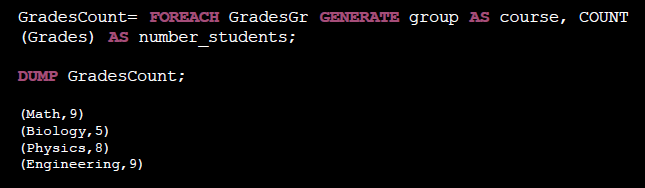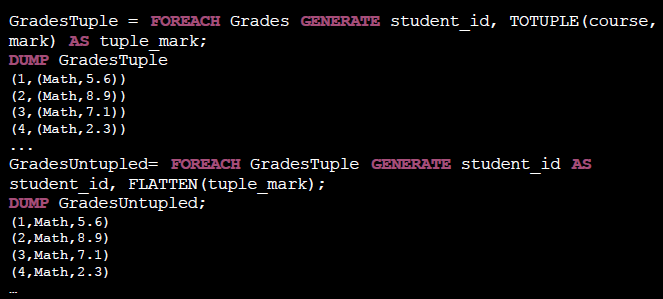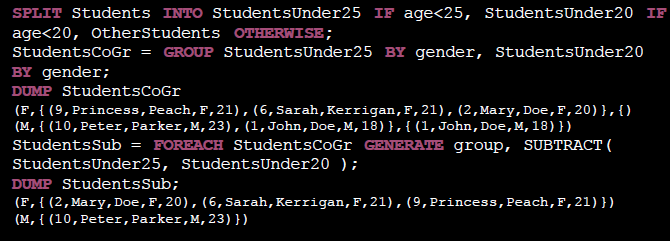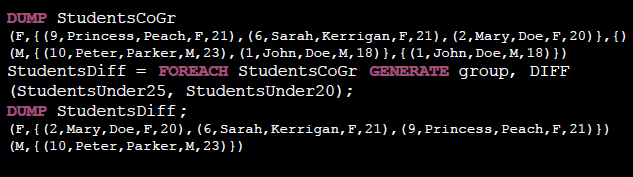# pig tutorial - apache pig tutorial - Pig tutorial - pig latin - apache pig - pig hadoop

## Functions & user defined functions

• Transform data in data projections
• Built-in functions:
• math functions, string functions, datetime functions, casting functions, etc.
• User defined functions:
• Our own functions written in Java, Python, Ruby, Javascript, etc.
• Bag functions:
• AVG/MAX/MIN/SUM: compute the average/max/min/sum of a bag of numeric values
•• COUNT: number of elements (not null) in a bag
•• Bag/Tuple functions:
• FLATTEN: behavior depends on input• SUBTRACT: Tuples on first bag not in the second• DIFF: Non overlapping tuples on two bags• Math functions:
• Common math functions for numeric values:
• ABS
• EXP
• FLOOR
• LOG
• RANDOM
• ROUND
• SQRT
• ...
• String functions:
• Transform chararrays:
• ENDSWITH
• LOWER
• UPPER
• SUBSTRING
• TRIM
• REPLACE
• ...
• Datetime functions:
• Get information on dates and timestamps: